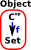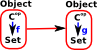# Maths - Presheaves

A 'presheaf' category is a special case of a functor category (see page here). It is a contravarient functor from a category 'C' to Set.

Since it is contravarient it is usually written:

CopSet

or

SetCop

## Presheaf CategoryIn a presheaf category the object is a functor.Morphisms are structure preserving maps between these functors.

In the theory of topological space a sheaf is a tool for systematically tracking locally defined data attached to the open sets of a topological space.

## Presheaf Examples

• A simplicial set is a presheaf on the simplex category
• A globular set is a presheaf on the globe category.
• A cubical set is a presheaf on the cube category.

### Example - Single Element Set

A very simple example would be where Cop is a single element set (terminal object in set).

Hom( Cop, Set) therefore contains set of single arrows, one for every element of the set.### Example - Graph

Here Cop is a category with two objects E (for edge) and V (for vertex) also two arrows s (for source) and t (for target).

This allows us to build a structure on top of set where the diagram on the right commutes.

We can therefore build up complex graphs from individual vertices and edges.### Example - Relational Database

Here Cop is a database schema.

This imposes a structure on the sets which are the database tables.

This implements a category of simplical databases.The following two videos discuss some of these concepts in a slightly topological way. For instance maps into sets related to fibres.

Where I can, I have put links to Amazon for books that are relevant to the subject, click on the appropriate country flag to get more details of the book or to buy it from them.The Princeton Companion to Mathematics - This is a big book that attempts to give a wide overview of the whole of mathematics, inevitably there are many things missing, but it gives a good insight into the history, concepts, branches, theorems and wider perspective of mathematics. It is well written and, if you are interested in maths, this is the type of book where you can open a page at random and find something interesting to read. To some extent it can be used as a reference book, although it doesn't have tables of formula for trig functions and so on, but where it is most useful is when you want to read about various topics to find out which topics are interesting and relevant to you.